### Floating Bodies Class 9th Physics & Chemistry AP Board Solution

##### Question 1.A solid sphere has a radius of 2 cm and a mass of 0.05 kg. What is the relative density of the sphere?Answer:As we all knew the density of an object is =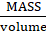,which has a unit of g/cm2.BUT the relative density is dimension less density which is define as =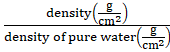So,Mass = 0.05 kg = 50 gradius = 2 cmvolume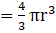cm3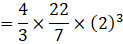=33.52cm3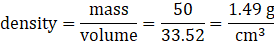density of water = 1 g/cm3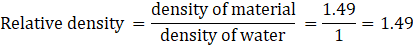So relative density of sphere is 1.49.Question 2.A small bottle weighs 20 g when empty and 22 g when filled with water. When it is filled with oil it weighs 21.76 g. What is the density of oil?Answer:Given that-Weight of empty bottle = 20 g ,And weight of water filled bottle = 22gSo the weight of water will be = 22-2 = 2g.As we knew density of water = 1 g/cm3.Then the volume of water will be =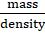= 2/1 = 2 cm3So capacity of bottle = 2cm3.So the volume of oil which is filled in bottle is = 2cm3.Also given that weight of bottle filled with oil = 21.76g.Weight of oil will be = 21.76 - 20 = 1.76gThen the density of oil =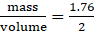= 0.88 g/cm3Question 3.An ice cube floats on the surface of a glass of water (density of ice =0.9 g/cm3 ). When the ice melts will the water level in the glass rise?Answer:By the Archimedes' principle When an ice block is floating on water, the amount of water displaced by the ice is equal to the mass of the ice. As we know that mass of ice is conserved. Ice and water both have same mass but have different volume .Thus when ice melts, the level of water will remain unchanged. This can also be observed for a boat on a pool. If you are on a boat, which is in a pol containing finite amount of water, if you fill up the boat with some water from the pool, the water level will be the same.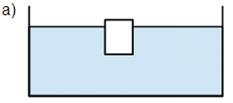Question 4.The volume of 50g of a substance is 20 cm3. If the density of water is 1g/cm3,will the substance sink or float when placed on the surface of water ? What will be the mass of water displaced by the substance?Answer:Given that Mass of substance is = 50g and volume is = 20cm3.Then the density of the substance will be =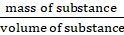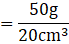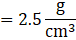As we know that density of water = 1 g/cm3According to Archimedes' principle the substances having higher density then water will sink in water & substances having density lower then water will float on the surface of water.Since the density of the substance is greater than the density of water, it will sink in water.The volume of water displaced =Volume of substance = 20 cm3Mass of water displaced By substance = volume × density = 20×1 = 20gQuestion 5.Find the pressure at a depth of 10m in water if the atmospheric pressure is 100kPa. [1Pa=1N/m2 ] [100kPa = 105Pa = 105N/m2 = 1 atm.]Answer:If we consider a tank of height h , filled with water then the pressure at the bottom surface of tank will be (look at the figure also)-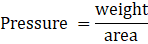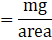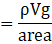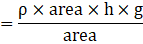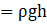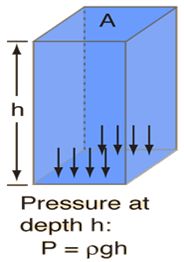As the pressure at the surface of water is atmospheric, soPressure at a depth h below the surface of water = atmospheric pressure + pressure due to water column of height h.Given that height of tank is h= 10mThe atmospheric pressure = 100 kPaDensity of water =1g/cm3 = 103 kg m–3The gravitational constant g = 10 m s–2Then the pressure at a depth h will be = atmospheric pressure + pressure at bottom= 100kpa +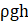= 105 N/m2 +103k/m3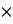10m/s210m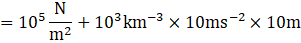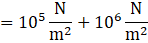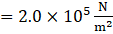= 200kpaQuestion 6.Why some objects float on the water? And some sink?Answer:According to Archimedes' principle Floating or sinking of an object does not depend on its weight (Mass), it depends upon its density. Things denser than water sink in water whereas things having less density than water float over it.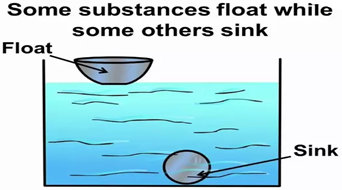Question 7.Explain density and relative density and write formulae.Answer:Density is the measure of mass per volume. This means that density of any solid,liquid, or gas can be found by dividing its mass in kilogram by its volume in cubic metres.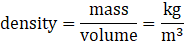the unit for density is Kg per metre cube. It can be expressed in g/cm3(ml)Relative density→ it is ratio of a density of the substance to the density of the given reference material. Lets assume our reference material is water then density relative to water will be -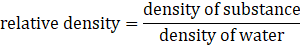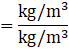Relative density is a ratio, it’s a dimensionless quantity.Question 8.What is the value of density of water?Answer:The density of water is the weight of the water per its unit volume, which depends on the temperature of the water. The usual value used in calculations is 1 gram per milliliter (1 g/ml) or 1 gram per cubic centimeter (1 g/cm3).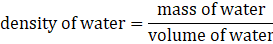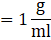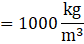Question 9.Find the relative density of wood. Explain the process.Answer:Relative density is also called specific gravity of a solid .Relative density can be determined using Archimedes principle.Arccoding to Archimedes principle ,when a body is wholly or partially immersed in a fluid ,it experience an upward thrust equal to the weight of the fluid displaced. Archimedes principal can be utilised to determine the relative density of solid substance that does not dissolve in water.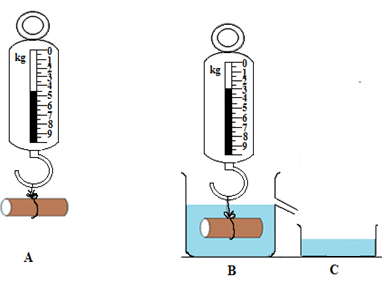As given in the figure first we measured the weight of the object in air which is given in A , then we put the system in the water vessel the weight the object in water(B) Is noted .As we put our system in the water vessel some amount of liquid will comes out from the vessel, which is collected in C . Now we can easily measure the weight of water in C. The data are following -A is a real weight (weight of an object in air) = W1B is apparent weight (weight of an object in fluid) = W2C is apparent loss of weight (weight of fluid displaced) = W1-W2Measuring the weight of displaced fluid is equal to apparent loss of weight; this apparent loss is the buoyancy force on an object which is upthrust.Apparent loss of weight is equal to the weight of displaced fluid.Real weight = apparent weight + apparent lossSince apparent loss is equal to upthrustReal weight = apparent weight + upthrustUpthrust =real weight – apparent weight.Now we can find the relative density of the substance ,which tells us how much the substance is denser then water.The formula for relative density is-The relative density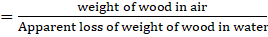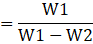Question 10.Which is denser, water or milk?Answer:Milk is denser then water. Milk is not just water. it is an emulsion of fats and proteins in water. Simply speaking, fat molecules along with proteins sort of hang on to water molecules to form a uniform mixture thereby increasing the density of the milk which is heavier than water.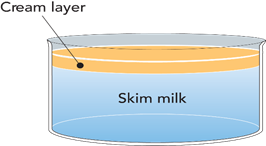Question 11.What is buoyancy?Answer:Arccoding to Archimedes principle ,when a body is wholly or partially immersed in a fluid ,it experience an upward thrust equal to the weight of the fluid displaced . This upward thrust is also known as Buoyancy.Every object experiences the upward thrust as it dipped in a liquid . The buoyancy force is the reason behind floating of objects .If the weight of volume displaced by object is more than the weight of the object ,then the object will float on the surface. And If the weight of volume displaced by object is less than the weight of the object.Then the object will sink in the liquid.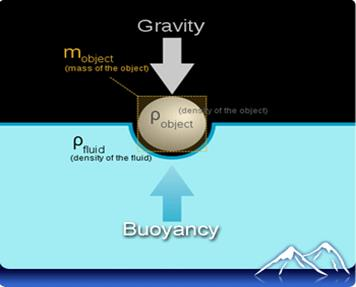If you look at the figure its clear in the figure that densities of object and liquid are different , so there exist a buoyancy force which is acts upward opposite in the direction of gravity.Question 12.Classify the following things into substances having RelativeDensity > 1 and Relative Density < 1 Wood, iron, rubber, plastic,glass, stone, cork, air, coal, ice, wax, paper, milk, kerosene,groundnut oil, soapAnswer:Relative density >1 , means the substance is denser than water and will sink in water.Relative density < 1, means the substance is less denser than water and will float on water.We can divide these objects by their floating properties(which we saw in our daily life)Substance having Relative density <1(Floating objects) : wood , plastic ,air ,rubber ,ice,wax,Kerosene, groundnut oil, soap.Substance having Relative density >1(sinking objects) : iron ,glass,stone.coal ,milk,paper.Question 13.How can you appreciate the technology of making ships float,using the material which sink in water?Answer:Floating and sinking is affected and determined by several factors that include:● density of material● shapeAs we know that If the weight of volume displaced by object is more than the weight of the object, then the object will float on the surface. And If the weight of volume displaced by object is less than the weight of the object,then the object will sink in the liquid. This is the main reason behind the floating of ships.The property that manufactures of ships use to make ships float on water, is by designing the right shape. The shape is designed in away that ensures that the amount of water that the ship displaces is just enough to get the ship submerged slightly.If the weight of liquid displaced by ship is more than the weight of ship then only the ship will float on water.Look at the figure ,it is clearly shown that Buoyancy force is enough to make the ship float on water.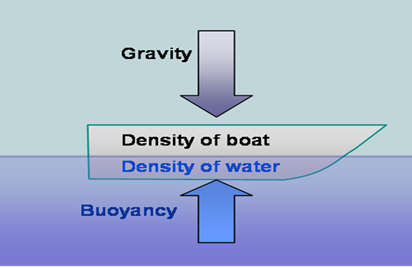Question 14.Can you make iron to float? How?Answer:YES , you can make iron to float , if its shape is such that the water desplaced by it has equal or more than weight of that iron . Many iron ships are there which floats on water.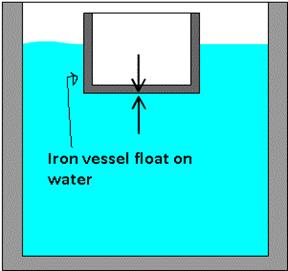As you can clearily see in the figure that the shape of iron veseel is such that the weight of water displaced is much more then the weight of iron vessel itself.EXAMPLE - An iron ship floats because it is shaped such that it will contain air within its hull and therefore the average density of the ship as a whole including cavities and all the air space is less than the water and therefore it floats.Question 15.How can you find the relative density of a liquid?Answer:As we know that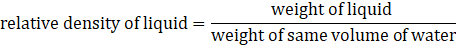There are some steps which we can follow for determination of relative density of the liquid, these are –● First we will take some water in a 50ml bottle and measured its weight (lets w1).● Measured the empty weight of the 50ml bottle (lets w2).● Now the weight of water will be = weight of bottle with liquid – weight of empty bottle=w1-w2.● Fill the bottle with some other liquid and measured its weight (w3).Now the weight of the liquid will be = weight of bottle with liquid –weight of empty bottle= w3-w2Then according to the relative density formula we have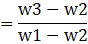Question 16.Find the relative density of different fruits and vegetables and write a listAnswer:RELATIVE Density is defined as ratio between density of object to the density of water.We can find out the different objects RELATIVE Density by an experiment and tabulate the results as follows: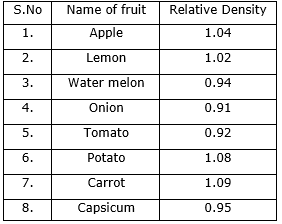Question 17.Make a lactometer with refill. What you do to make the refill stand vertically straight?Answer:Lactometer is an instrument which is used to test the purity of milk.It Works on Archimedes PrincipleTO make a lactometer with ball point refill, follow the given steps:● Take an empty ball pen refill.● Refill should have a metal point at the bottom.● Take a boiling tube and fill it with water.● Put the refill in the water with metallic point.● To make the refill stand vertically in water, insert a small eraser piece in the refill at the centre.● Mark the point with marker up to which the refill is above the water.● Now, empty the boiling tube with water and fill it with milk.● Again put the refill in milk and mark the point which shows the part which is above the surface of milk.● Further we can add , milk water mixture in the boiling tube and repeat the same step 8.● we can now compare and test whether water is added to milk or not.Look at the two figure given below ,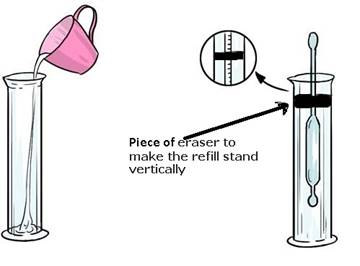LACTOMETER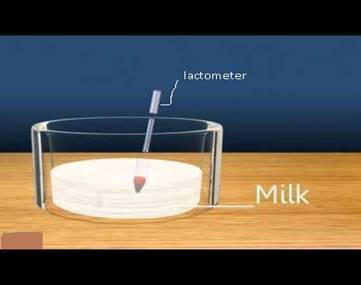Question 18.Draw the diagram of mercury barometer.Answer: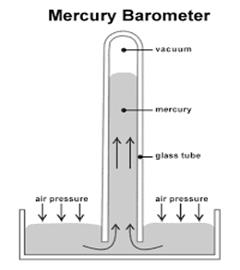Mercury Barometer (1)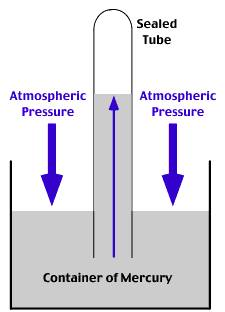Mercury Barometer (2)Question 19.How do you appreciate Pascal’s discovery in helping to makehydraulic jacks.Answer:Hydraulic jacks-The hydraulic jack is a device used for lifting heavy loads by the application of much smaller force. It is based on Pascal’s law. The Pascal's law states that the pressure at a point in a fluid at rest is the same in all directions.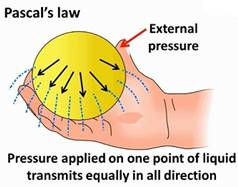WORKING PRINCIPLE:The working principle of a hydraulic jack may be explained with the help of Figure Consider two piston with different surface area A1 & A2, operating in two cylinders of different diameters, which are interconnected at the bottom, through a chamber, which is filled with some liquid.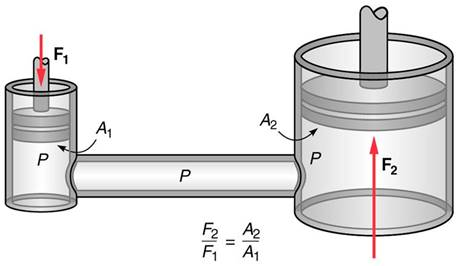LetW= Weight to be lifted,F1 = Force applied on piston 1,A1 = Area piston 1.A2 = Area of piston2.F2 = Force applied on piston 2 = W.Pressure intensity produced by the force F1,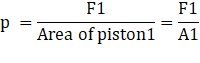As per Pascal’s law, the above intensity p will be equally transmitted in all directions.Therefore, The pressure intensity on Piston2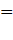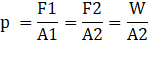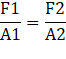Above Equation indicates that by applying a small force F1 on the Piston1, a large force W or F2 may be developed by the Piston2.Hydraulic jack have many uses in our life some of them are following -● It is used in Metal press work to press sheet metal to any required shape by applying pressure on it .● As it can produce large force from small forces ,I can be used in Drawing and pushing rods.● It can Bend and straight any metal piece.● Many Packing press are uses hydraulic jack.● Lifting up heavy cars .Question 20.How do you appreciate Archimedes discovery of force of buoyancy.Answer:Archimedes’ principle, physical law of buoyancy, discovered by the ancient Greek mathematician and inventor Archimedes, stating that any body completely or partially submerged in a fluid(gas or liquid) at rest is acted upon by an upward, or buoyant, force the magnitude of which is equal to the weight of the fluid displaced by the body.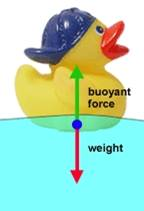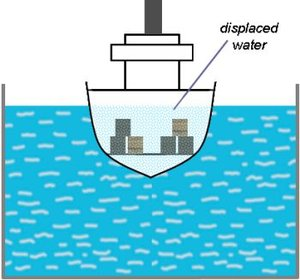There are many things in our day-to day life in which we can appreciate Archimedes discovery of buoyancy:-● It keeps all very heavy sea craft and ships afloat to bring all your comforts from overseas across the oceans.● It helps you to float when you go to swim and it is also used in lifejackets.● IN the past it helped airships to fly.● It helps to make float glass so beautifully flat .● It helps the solids to go down the drainpipes away from our homes and so we keep healthy.● It helps to release gas in our stomach and take the bubbles out of fizzy lemonade.● It helps the clothes in the washing machines not to accumulate at the bottom, and to become lighter and so they circle around in the water much easier to get clean.● Buoyancy can help to make oil water separators.● It helps to make the winds and the weather as hot air rises due to its buoyancy but can turn into a tornado if it cools down.Question 21.You found the relative densities of some solids and some liquids by some activities. List the solids and liquids in increasing order of relative densityAnswer: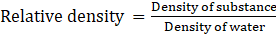.Relative densities of Some solids :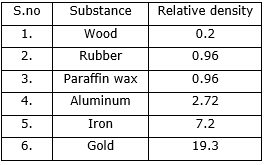Relative density Of some liquids :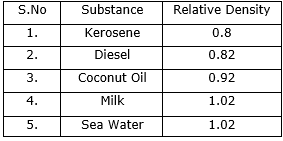Question 22.Iron sinks in water, wood floats in water. If we tied an iron piece to the same volume of wood piece, and dropped them in water, will it sink or float? Make a guess and find out whether your guess is correct or wrong with an experiment. Give reasons.Answer:If we tied the a piece of iron on wood , but the wood shape is such that the weight of combined system (iron +wood) is less than the water displaced by wood , then only the combined system will float on water. Otherwise the (wood+iron) will sink.Arccoding to Archimedes principle ,when a body is wholly or partially immersed in a fluid ,it experience an upward thrust equal to the weight of the fluid displaced. If the weight of fluid displaced is greater than the weight of substance than the substance will float on liquid.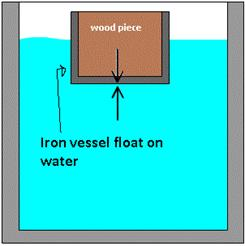As shown in figure if the (wood+ iron) displaced more weight of water then itself then it can float on water surface.Question 23.Liquid brakes in automobiles follow principle of bramha press (Pascal’s principle). What about air brakes? Collect the information about the working process of air brakes.Answer:The working of air brakes uses many component system like engine , compressor ,Storage tank, Main Control Device ,Brake Chamber & Drum Brake.● First of all the compressor takes the power from the engine to compress the atmospheric air.● After air compression the air is stored in storage tank. It also regulates the pressure of the tank.● There exist a main control Device which regulates the pressure of air which allows to change the brake intensity.● After this the Brake chamber is their which basically converts air pressure into mechanical force. It act as return device when brake is released.● Then finally the Drum Brake creates friction ,which stops the wheels of the vehicle.The layout of air break is given in the figure.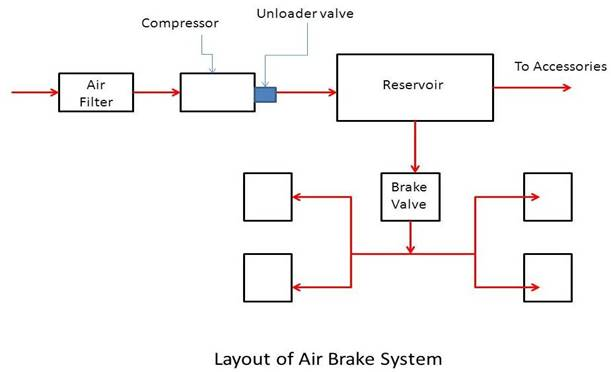Question 24.Where do you observe Archimedes principle in our daily life? Give two examples.Answer:There are many things in our daily life in which we see Archimedes principle some of them are –● The mug floats when we try to push it in in water.● Grains sink and soak water then start floating.● When we put a plastic ship it float on water.● Air jackets/air balls float in swimming pools.Question 25.Where do you observe Pascal’s principle in our daily life? Give few examples.Answer:Pascal principle: it states that when the force applied on particle of fluid the intensity of pressure force is equal in all directions.Now the uses of Pascal's principle● it is used in Hydraulic Jack● it is used in Hydraulic brakes in car● hydraulic lifts.● Filling balloons with air.● In water shower in bathrooms.

PDF FILE TO YOUR EMAIL IMMEDIATELY PURCHASE NOTES & PAPER SOLUTION. @ Rs. 50/- each (GST extra)

HINDI ENTIRE PAPER SOLUTION

MARATHI PAPER SOLUTION

SSC MATHS I PAPER SOLUTION

SSC MATHS II PAPER SOLUTION

SSC SCIENCE I PAPER SOLUTION

SSC SCIENCE II PAPER SOLUTION

SSC ENGLISH PAPER SOLUTION

SSC & HSC ENGLISH WRITING SKILL

HSC ACCOUNTS NOTES

HSC OCM NOTES

HSC ECONOMICS NOTES

HSC SECRETARIAL PRACTICE NOTES

# 2019 Board Paper Solution

HSC ENGLISH SET A 2019 21st February, 2019

HSC ENGLISH SET B 2019 21st February, 2019

HSC ENGLISH SET C 2019 21st February, 2019

HSC ENGLISH SET D 2019 21st February, 2019

SECRETARIAL PRACTICE (S.P) 2019 25th February, 2019

HSC XII PHYSICS 2019 25th February, 2019

CHEMISTRY XII HSC SOLUTION 27th, February, 2019

OCM PAPER SOLUTION 2019 27th, February, 2019

HSC MATHS PAPER SOLUTION COMMERCE, 2nd March, 2019

HSC MATHS PAPER SOLUTION SCIENCE 2nd, March, 2019

SSC ENGLISH STD 10 5TH MARCH, 2019.

HSC XII ACCOUNTS 2019 6th March, 2019

HSC XII BIOLOGY 2019 6TH March, 2019

HSC XII ECONOMICS 9Th March 2019

SSC Maths I March 2019 Solution 10th Standard11th, March, 2019

SSC MATHS II MARCH 2019 SOLUTION 10TH STD.13th March, 2019

SSC SCIENCE I MARCH 2019 SOLUTION 10TH STD. 15th March, 2019.

SSC SCIENCE II MARCH 2019 SOLUTION 10TH STD. 18th March, 2019.

SSC SOCIAL SCIENCE I MARCH 2019 SOLUTION20th March, 2019

SSC SOCIAL SCIENCE II MARCH 2019 SOLUTION, 22nd March, 2019

XII CBSE - BOARD - MARCH - 2019 ENGLISH - QP + SOLUTIONS, 2nd March, 2019

# HSCMaharashtraBoardPapers2020

(Std 12th English Medium)

HSC ECONOMICS MARCH 2020

HSC OCM MARCH 2020

HSC ACCOUNTS MARCH 2020

HSC S.P. MARCH 2020

HSC ENGLISH MARCH 2020

HSC HINDI MARCH 2020

HSC MARATHI MARCH 2020

HSC MATHS MARCH 2020

# SSCMaharashtraBoardPapers2020

(Std 10th English Medium)

English MARCH 2020

HindI MARCH 2020

Hindi (Composite) MARCH 2020

Marathi MARCH 2020

Mathematics (Paper 1) MARCH 2020

Mathematics (Paper 2) MARCH 2020

Sanskrit MARCH 2020

Sanskrit (Composite) MARCH 2020

Science (Paper 1) MARCH 2020

Science (Paper 2)

Geography Model Set 1 2020-2021

MUST REMEMBER THINGS on the day of Exam

Are you prepared? for English Grammar in Board Exam.

Paper Presentation In Board Exam

How to Score Good Marks in SSC Board Exams

Tips To Score More Than 90% Marks In 12th Board Exam

How to write English exams?

How to prepare for board exam when less time is left

How to memorise what you learn for board exam

No. 1 Simple Hack, you can try out, in preparing for Board Exam

How to Study for CBSE Class 10 Board Exams Subject Wise Tips?

JEE Main 2020 Registration Process – Exam Pattern & Important Dates

NEET UG 2020 Registration Process Exam Pattern & Important Dates

How can One Prepare for two Competitive Exams at the same time?

8 Proven Tips to Handle Anxiety before Exams!